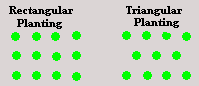Spike's Calculators

# Plant Spacing MultiplierFind the multiplier for number of plants needed based on rectangular and triangular pattern planting.

This multiplier can be used to estimate the amount of plants needed for an area.

##### Example:
```Enter the amount of spacing required in the calculator.
Required plant spacing = 14"
The multiplier for 14" plant spacing for rectangular pattern planting = 0.73
The multiplier for 14" plant spacing for triangular pattern planting =  0.85

An area of 20 feet by 30 feet for planting.
Square footage of area to be planted = 20' x 30' = 600 sq feet

Multiply your square footage by the multiplier.
Estimated amount of plants needed for rectangular pattern planting 600 x 0.73 =  438 plants.
Estimated amount of plants needed for triangular pattern planting 600 x 0.85 =   520 plants.
```
On centre spacing: inch

#### Results:

 Square Pattern Planting: # Triangular Pattern Planting: #